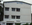Trending ▼   ResFinder# ICSE Prelims 2017 : Economic Applications (International Public School, Michael Nagar, Jessore Road, Kolkata)

2 pages, 34 questions, 1 questions with responses, 1 total responses,00Good Lode International Public School, Michael Nagar, Jessore Road, Kolkata
+Fave Message
 Home > thakurdas >   F Also featured on: School PageFormatting page ...

Class 10 time 2 hours full marks 100 Attempt all questions from PART I and any four from PART II. The intended marks of questions are given in brackets ( ) PART I (40 MARKS) QUESTION 1. Explain the following terms: 5x2=10 a. Ceteris paribus assumption of the law of demand. b. Sunk capital. c. Monetary policy. d. Product differentiation. e. Cash reserve ratio. QUESTION 2. State whether the following statements are true or false. Give reasons. 5x2=10 a. The price elasticity of demand for commodities having close substitutes is relatively low. b. The price level in a perfectly competitive market is determined by a group of individual seller. c. Efficiency of labour is influenced by working conditions. d. Direct taxes are economical. e. Commercial banks do not promotes economical development. QUESTION 3. 5x2=10 a. Draw a market supply curve from a hypothetical supply schedule. b. With the help of diagram show the effect of left ward shift of demand curve, with proper levelling. c. State any two adverse effect of change in patterns of land use. d. The supply of labour in a country depends on three factors . What are the factors? e. What are the three important functions of an entrepreneur? QUESTION 4. 5x2=10 a. Write four features of monopolistically competitive market. b. what do you mean standard of deferred payment . c. What is stagflation? d. What is repo rate ? e. Explain moral suasion of RBI. PART II ( 60 MARKS) ATTEMPT ANY FOUR QUESTIONS. QUESTION 5. 7+8=15 a. What are the role of banks in an economy? b. What are the quantitative method of credit control? QUESTION 6. a. What are the effect of inflation on an economy? b. What was/ is the advantages of privatisation in Indian economy? QUESTION 7. a. What are the objectives of fiscal policy? b. What are the general reasons for the emergence of monopoly market structure. QUESTION 8. a. What are the characteristics of entrepreneur? b. What are the advantages of division of labour? QUESTION 9. a. Define price elasticity of supply. With the help of suitable diagram, explain the following degrees of supply elasticity. i. Elastic supply curve, ii. Inelastic supply curve. b. Define composite demand. Explain any three factors determine demand in the market.Formatting page ...

Top Contributors
to this ResPaperIcse(1)